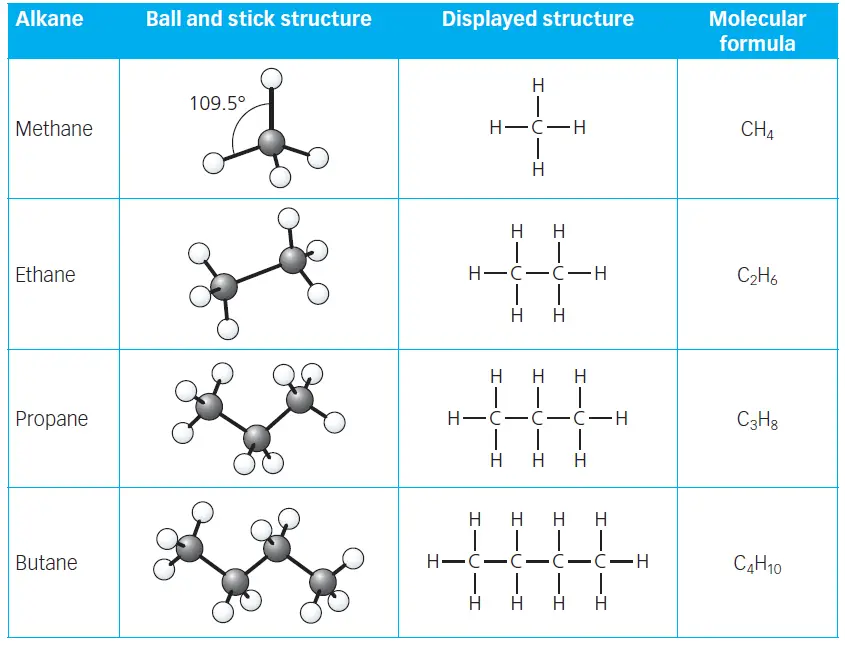# Alkanes & The first four alkanes – revision notes

Alkanes are a family of saturated hydrocarbons. Saturated molecules are ones that only contain single covalent bonds. The structures of the first four alkanes are shown in table 1. The table includes the displayed formula which shows all the atoms and all the bonds in each molecule.

## The first four alkanes

The first four alkanes are Methane, Ethane, Propane, and Butane.

The following table shows the Ball and stick structure, displayed formula, and molecular formula of the first four alkanes.table 1: Ball and stick structure, displayed structure, and the molecular formula of the first four alkanes.

All the alkanes have a molecular formula of the form CnH2n+2. For example, if there are 3 carbon atoms (n = 3), then there are 8 hydrogen atoms (2n + 2 = (2 × 3) + 2 = 8). This and other examples are shown in Table 2.